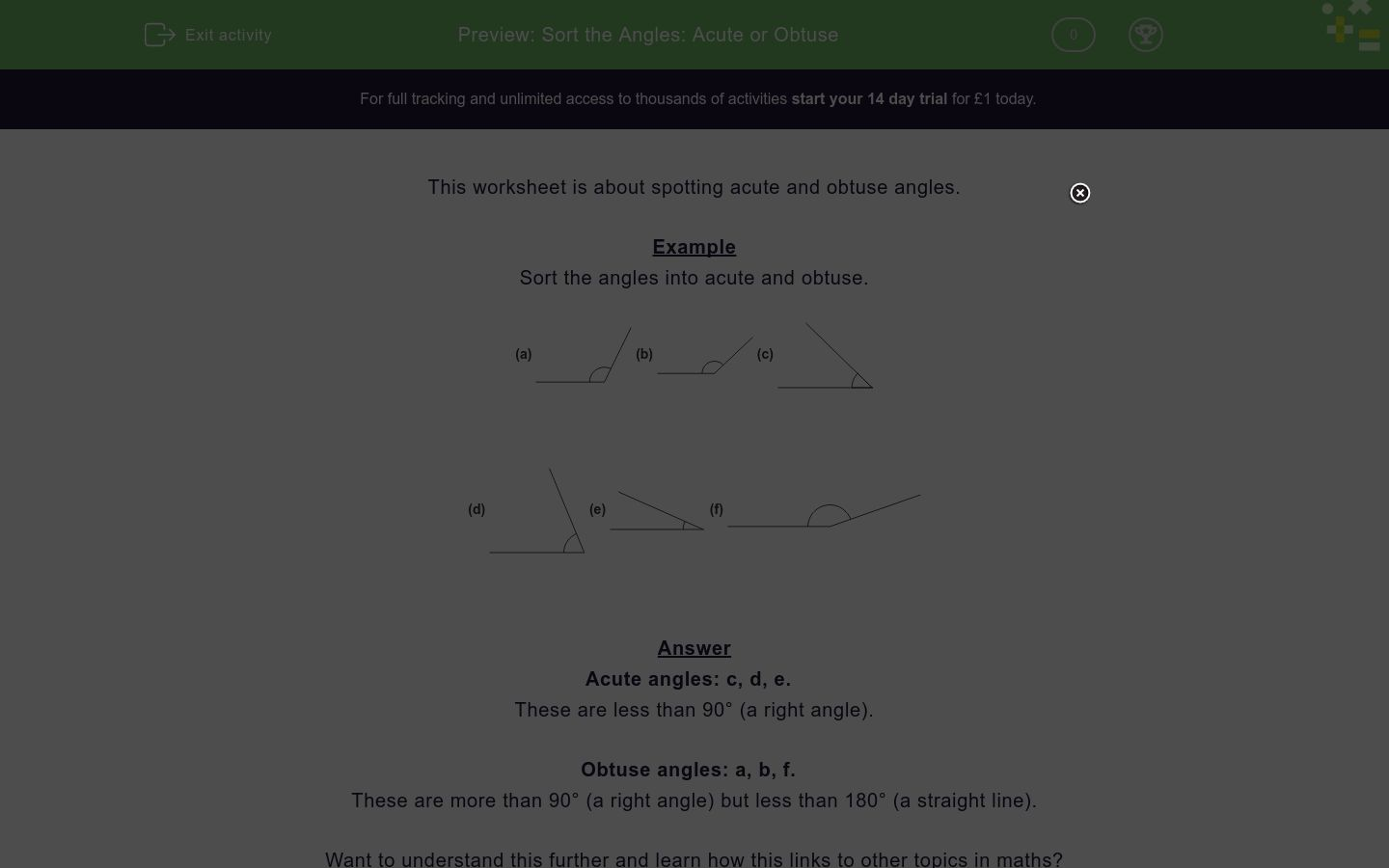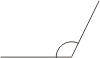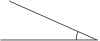# Sort the Angles: Acute or Obtuse

In this worksheet, students sort angles into acute or obtuse.Key stage:  KS 2

Curriculum topic:   Geometry: Properties of Shapes

Curriculum subtopic:   Identify Acute/Obtuse Angles

Difficulty level:### QUESTION 1 of 10

This worksheet is about spotting acute and obtuse angles.

Example

Sort the angles into acute and obtuse.

(a)(b)(c)(d)(e)(f)Acute angles: c, d, e.

These are less than 90° (a right angle).

Obtuse angles: a, b, f.

These are more than 90° (a right angle) but less than 180° (a straight line).

Want to understand this further and learn how this links to other topics in maths?
Why not watch this video?

---- OR ----